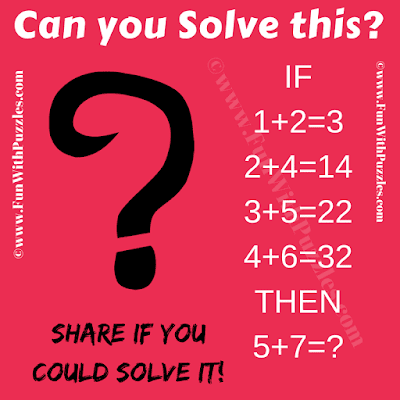## Tuesday, December 18, 2018

This Tricky Logic Riddle is for Teens. Even though this Logic Riddle is not very tough but Kids will find this Logic Riddle very tricky. There is simple logic hidden in this Riddle. Can you decipher the hidden logic and solve this Tricky Logic Riddle?Can you solve this Tricky Logic Riddle?

1.i have found the logic, a+b= b^2-a

1.Great. Your answer and explanation to this tricky logic #riddle is correct.

2.If 1+2=3
2×4+4+2=14
2+4=14
3×5+5+2=22
3+5=22
4×6+6+2
4+6=32
5×7+7+2=44
5+7=44

1.Your answer to this tricky logic riddle is right. However, your logical reasoning used to solve this #riddle is different from our reasoning. Your logical reasoning does not work on the 1st equation.
Thanks for your detailed comment. I will create a puzzle on this logical reasoning soon.

A comment doesn't cost a thing. Please drop a comment below to boost the author's morale.